## Incompressible Mathematical Model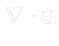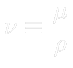• Mass conservation• Momentum conservation• where: Symbol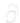is partial derivative,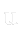is velocity vector,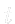is time,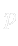is static pressure [Pa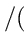kg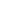m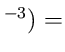/"/” />m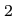s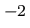],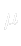is dynamic viscosity,is kinematic viscosity and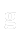is gravity force vector.
• The whole system is closed with boundary conditions.